Latest Banking jobs   »   Quantitative Aptitude Quiz For ESIC- UDC,...

# Quantitative Aptitude Quiz For ESIC- UDC, Steno, MTS Prelims 2022-26th January

Q1. Simple interest on a certain sum at the rate of 16⅔% per annum for three years is Rs. 1250. Find the sum.
(a) Rs. 3,000
(b) Rs. 2,500
(c) Rs. 2,400
(d) Rs. 4,000
(e) Rs. 5,000

Q2. A fraction becomes 5/3 when 20% of numerator is added to its numerator and 30% of denominator is subtracted from its denominator. Find the fraction.
(a) 35/36
(b) 36/25
(c) 33/35
(d) 27/35
(e) 35/33

Q3. Rajjo borrows Rs. 6300 from PNB on compound intrest at the rate of 33⅓% p.a. for two years. Find the total interest given by Rajjo to the bank after 2 years
(a) Rs.4,100
(b) Rs.3,900
(c) Rs.4,900
(d) Rs.4,600
(e) Rs. 4,500

Q4. Raheem spends 36% of his monthly income on daily spendings, 40% on house rent and children fee together and rest amount he saves for future needs. If his total savings be Rs. 14,400. Find his total monthly income.
(a) Rs. 45,000
(b) Rs. 40,000
(c) Rs. 48,000
(d) Rs. 60,000
(e) Rs. 55,000

Q5. The population a city after three years will be 21,600. If rate of increase of population per year be 20% then find the present population of the city.
(a) 12,500
(b) 16,500
(c) 14,500
(d) 10,500
(e) 11,600

Directions (6-10): Solve the following questions and find the value of (?)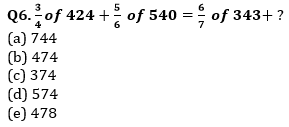Q7. 33% of 600 + 44% of 225=? % of 500
(a) 56.4
(b) 5.94
(c) 59.4
(d) 49.4
(e) 69.4

Q8. 3453 ÷ 30 + 5555 ÷ 500 =? – 777 ÷ 70
(a) 13.371
(b) 133.71
(c) 13.731
(d) 137.31
(e) 131.73

Q9. 101 × 6 + 450 ÷ 15 =? % of 200
(a) 813
(b) 318
(c) 418
(d) 518
(e) 218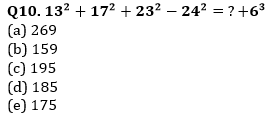Directions (11-15): Study the following line graph carefully and answer the following questions.
The line graph shows the no. of questions asked from different topics of quantitative aptitude in two shifts of SBI clerk prelims exam.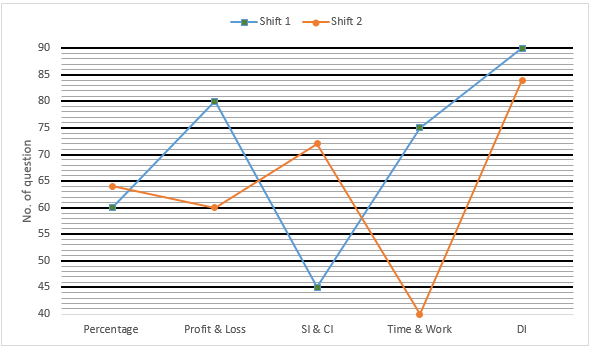Q11. No. of questions asked in shift 1 from SI & CI is what percent of no. of questions asked from time and work in the same shift?
(a) 60%
(b) 50%
(c) 55%
(d) 64%
(e) 75%

Q12. Find the average no. of questions asked from all the given sections in shift 2.
(a) 46
(b) 64
(c) 48
(d) 68
(e) 74

Q13. What is the ratio of no. of questions asked from profit & loss and percentage together in shift 1 to the questions asked from profit & loss and time & work together in shift-2?
(a) 6 : 5
(b) 5 : 7
(c) 7 : 5
(d) 5 : 6
(e) 8 : 7

Q14. What is the difference between total no. of questions asked in both shifts from all the given sections together?
(a) 45
(b) 40
(c) 35
(d) 30
(e) 60

Q15. Total no. of questions asked from DI in shift 2 are what percent less than that of DI in shift 1?
(a) 5⅔%
(b) 6⅔%
(c) 4⅔%
(d) 8%
(e) 3⅔%

Solutions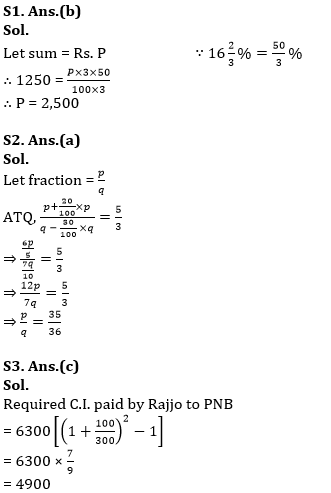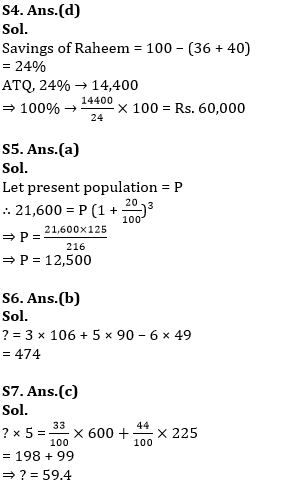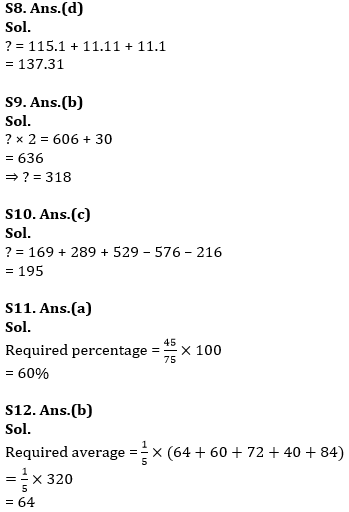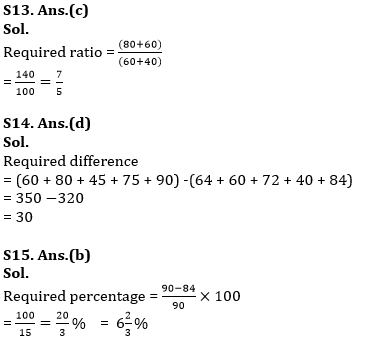#### Congratulations!